Maths-
General
Easy

Question

# If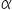and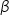are the eccentric angles of extremities of a focal chord of an ellipse, then the eccentricity of the ellipse is -

## The correct answer is: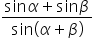### Equation of chord be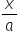cos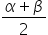+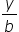sin= cos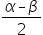since it passes through (ae, 0)so e cos= cose =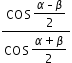=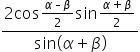e =#### With Turito Foundation.#### Get an Expert Advice From Turito.